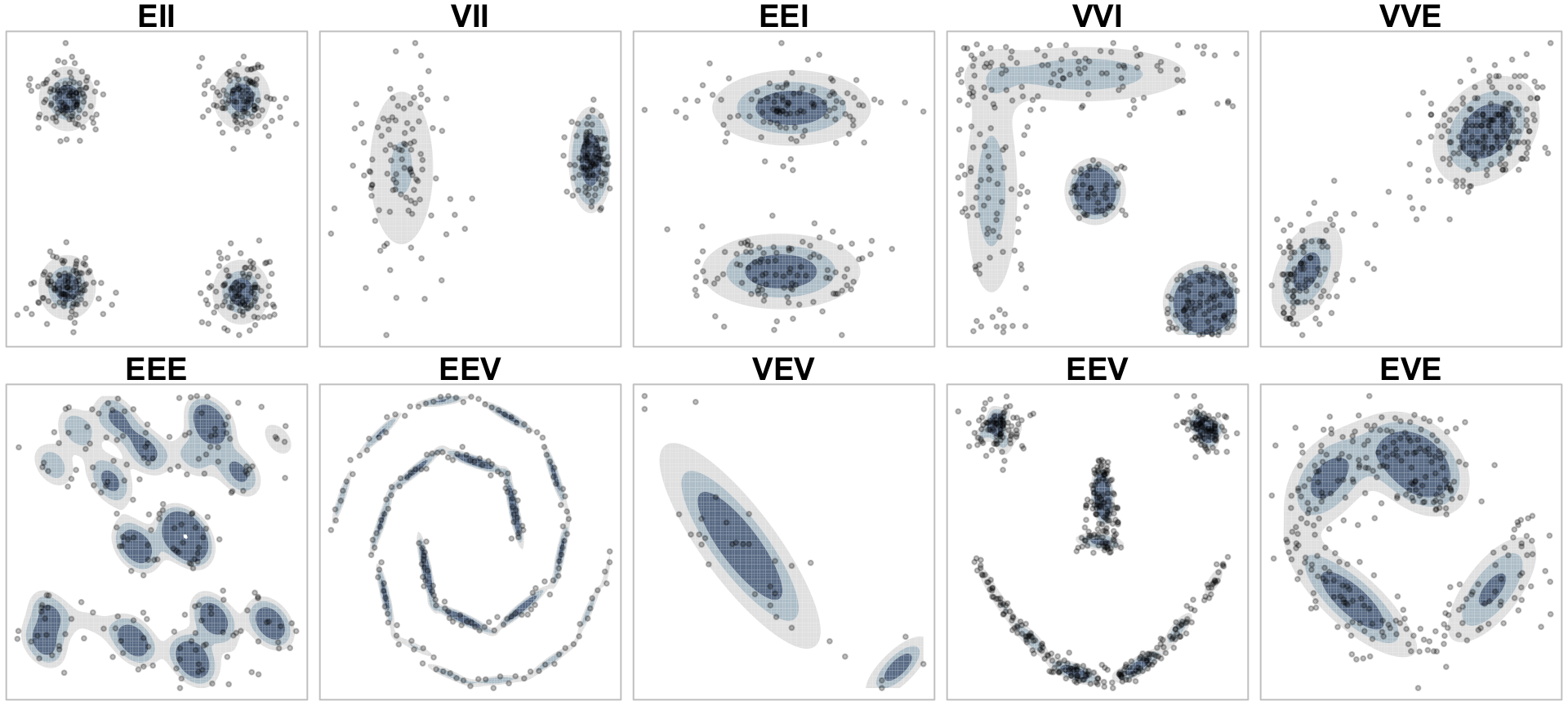--- title: "ETC3250/5250: Introduction to Machine Learning" subtitle: "Model-based clustering" author: "Professor Di Cook" email: "ETC3250.Clayton-x@monash.edu" date: "Week 11a" length: "50 minutes" department: "Department of Econometrics and Business Statistics" titlebgimg: "images/bg-02.png" output: xaringan::moon_reader: css: - ninjutsu - "assets/font-awesome-all.css" - "assets/tachyons-addon.css" - "assets/animate.css" - "assets/fira-code.css" - "assets/boxes.css" - "assets/table.css" - "assets/styles.css" - "assets/monash-brand.css" - "assets/monash-fonts.css" - "assets/slide-types.css" - "assets/custom.css" - "assets/panelset.css" self_contained: false seal: false chakra: 'lib/remark-latest.min.js' includes: in_header: "assets/head.html" lib_dir: lib #includes: # in_header: "assets/custom.html" mathjax: "lib/mathjax-local/MathJax.js?config=TeX-AMS-MML_HTMLorMML" nature: highlightStyle: magula highlightLanguage: r highlightLines: true highlightSpans: false countIncrementalSlides: false slideNumberFormat: '%current%/%total%' navigation: scroll: false touch: true click: false ratio: '16:9' --- {r, include = FALSE} current_file <- knitr::current_input() basename <- gsub(".Rmd$", "", current_file) knitr::opts_chunk$set( fig.path = sprintf("images/%s/", basename), fig.width = 6, fig.height = 4, out.width = "100%", fig.align = "center", fig.retina = 5, echo = FALSE, warning = FALSE, message = FALSE, cache = FALSE, cache.path = "cache/" )  {r titleslide, child="assets/titleslide.Rmd"}  {r} library(tidyverse) library(tourr) library(ggdendro) library(patchwork) library(GGally) library(kableExtra) library(yardstick) library(mclust)  --- # Overview Model-based clustering makes an assumption about the distribution of the data, primarily - Assumes the data is a sample from a Gaussian mixture model - Requires the assumption that clusters have an elliptical shape - .monash-orange2[The shape is determined by the variance-covariance of the clusters] - .monash-orange2[A variety of models is available by using different constraints on the variance-covariance] Model is $$f(x_i) = \sum_{k=1}^G\pi_kf_k(x_i; \mu_k, \Sigma_k)$$ where $f_k$ is usually a multivariate normal distribution. The parameters are estimated by maximum likelihood, and choice between models is made using BIC. ---Source: [Boehmke (2020) Hands-on machine learning](https://bradleyboehmke.github.io/HOML/model-clustering.html) --- # Variance-covariance specification Constraints applied on cluster variance-covariance: 1. .monash-blue2[volume]: each cluster has approximately the same size 2. .monash-blue2[shape]: each cluster has approximately the same variance so that the distribution is spherical 3. .monash-blue2[orientation]: each cluster is forced to be axis-aligned --- # Variance-covariance contraints |Model|Family|Volume|Shape|Orientation|Identifier| |---|---|---|---|---|---| |1|Spherical|Equal|Equal|NA|EII| |2|Spherical|Variable|Equal|NA|VII| |3|Diagonal|Equal|Equal|Axes|EEI| |6|Diagonal|Variable|Variable|Axes|VVI| |7|General|Equal|Equal|Equal|EEE| |8|General|Equal|Variable|Equal|EVE| |10|General|Variable|Variable|Equal|VVE| |11|General|Equal|Equal|Variable|EEV| |12|General|Variable|Equal|Variable|VEV| |14|General|Variable|Variable|Variable|VVV| --- class: split-33 .column[.pad50px[ # Example: nuisance variable {r out.width="80%", fig.width=4, fig.height=4} set.seed(20190512) df <- data.frame(x1=scale(c(rnorm(50, -4), rnorm(50, 4))), x2=scale(c(rnorm(100)))) ggplot(data=df, aes(x1, x2)) + geom_point() + theme_bw() + theme(aspect.ratio=1)  ]] .column[.content.vmiddle[ {r echo=TRUE} df_mc <- Mclust(df, G = 2) summary(df_mc)  ]] --- class: split-two .column[.pad50px[ {r fig.height=4, fig.width=4, echo=TRUE} plot(df_mc, what = "density")  ]] .column[.pad50px[ {r fig.height=4, fig.width=4, echo=TRUE} plot(df_mc, what = "uncertainty")  ]] --- class: split-two # Parameter estimates .column[.pad50px[

Cluster means {r echo=TRUE} options(digits=2) df_mc$parameters$mean  ]] .column[.pad50px[ Cluster variances {r echo=TRUE} df_mc$parameters$variance$sigma  ]] --- class: split-33 .column[.pad50px[ # Example: nuisance observations {r out.width="80%", fig.width=4, fig.height=4} set.seed(20190514) x <- (runif(20)-0.5)*4 y <- x df <- data.frame(x1=scale(c(rnorm(50, -3), rnorm(50, 3), x)), x2=scale(c(rnorm(50, -3), rnorm(50, 3), y))) ggplot(data=df, aes(x1, x2)) + geom_point() + theme_bw() + theme(aspect.ratio=1)  ]] .column[.content.vmiddle[ {r echo=TRUE} df_mc <- Mclust(df, G = 2) summary(df_mc)  ]] --- class: split-two .column[.pad50px[ {r echo=TRUE, fig.width=4, fig.height=4} plot(df_mc, what = "density")  ]] .column[.pad50px[ {r echo=TRUE, fig.width=4, fig.height=4} plot(df_mc, what = "uncertainty")  ]] --- class: split-two # Parameter estimates .column[.pad50px[ Cluster means {r echo=TRUE} df_mc$parameters$mean  ]] .column[.pad50px[ Cluster variances {r echo=TRUE} df_mc$parameters$variance$sigma  ]] --- class: split-66 .column[.pad50px[ {r echo=TRUE} set.seed(6) data(flea) flea_mc <- Mclust(flea[,2:7]) summary(flea_mc)  ]] .column[.content.vmiddle[ # Example: flea with nuisance variables and observations Let model-based decide number of clusters, and variance-covariance parametrization. {r out.width="80%", fig.width=4, fig.height=4} load(here::here("data/fproj.rda")) fproj <- fproj %>% mutate(cl = factor(flea_mc$classification)) ggplot(fproj, aes(x=V1, y=V2, colour=cl)) + geom_point() + scale_colour_brewer("", palette="Dark2") + theme_minimal(base_size = 18) + theme(aspect.ratio=1) + xlab("") + ylab("")  ]] --- class: split-two .column[.pad50px[ {r} plot(flea_mc, "BIC")  - The spherical models are clearly inferior. - Across many models, three clusters is where ther is a peak in BIC - A few parametrisations are nearly equally as good, pick the simplest. ]] .column[.pad50px[ {r out.width="100%", fig.width=8, fig.height=8} plot(flea_mc, "uncertainty")  ]] --- class: split-two # Parameter estimates .column[.pad50px[ Cluster means {r echo=TRUE} flea_mc$parameters$mean  ]] .column[.pad50px[ Cluster variances .scroll-800[ {r echo=TRUE} flea_mc$parameters$variance$sigma[,,1]  ] ]] --- # Summary - Model-based clustering provides a nice automated clustering, if the data has neatly separated clusters, even in the presence of nuisance variables. - Non-elliptical clusters could be modeled by combining multiple ellipses. - It is affected by nuisance observations, and has a parameter noise to attempt to filter these. - It may not function so well if the data hasn't got separated clusters. - k-means and Wards linkage hierarchical would yield similar results to constraining the variance-covariance model to EEI (or VII, EEE). - Having a functional model for the clusters is useful. --- {r endslide, child="assets/endslide.Rmd"}